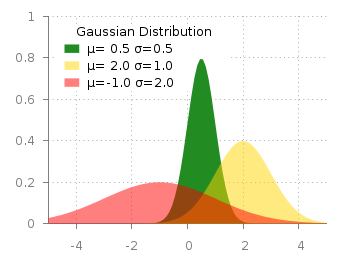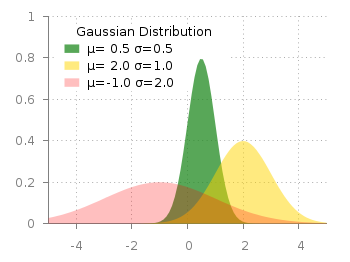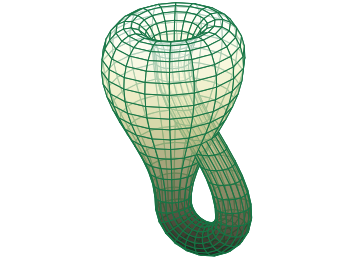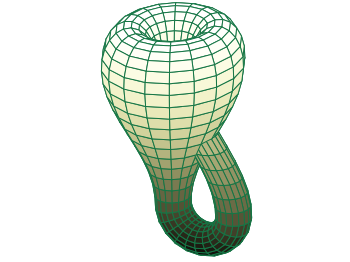# Gnuplotting

Create scientific plots using gnuplot

February 6th, 2013 | 8 Comments

Maybe you all know the nice example of gnuplots transparent fill style. I have replotted it slightly modified in Fig. 1.Fig. 1 Filledcurves with transparency settings as on the gnuplot demo site (code to reproduce this figure)

The interesting part in the code looks like this.

```set style fill transparent solid 0.5 noborder
plot d1(x) fs solid 1.0 lc rgb "forest-green" title 'µ= 0.5 σ=0.5', \
d2(x) lc rgb "gold" title 'µ= 2.0 σ=1.0', \
d3(x) lc rgb "red" title 'µ=-1.0 σ=2.0'
```

The set style command sets the fill style to 50% transparency, which is overwritten by the explicit fs option to the first plotting command in order to plot the green curve without transparency.Fig. 2 Filledcurves with different transparency settings (code to reproduce this figure)

Now the question is how to plot filled curves with different transparency settings? The simple answer is, by just using this explicit fs plot argument. The result is shown in Fig.2 and can be reached with the following code. Now we apply a transparency of 75%, 50%, and 25% going from the green to the red curve.

```set style fill noborder
plot d1(x) fs transparent solid 0.75 lc rgb "forest-green" \
title 'µ= 0.5σ=0.5', \
d2(x) fs transparent solid 0.50 lc rgb "gold" \
title 'µ= 2.0 σ=1.0', \
d3(x) fs transparent solid 0.25 lc rgb "red" \
title 'µ=-1.0 σ=2.0'
```

June 7th, 2011 | 6 Comments

A Klein bottle is a non-orientable surface, which has no defined left and right, as stated on Wikipedia. There we can also find a Gnuplot plot of the bottle, which we want to fine-tune a little bit in order to reach the result in Fig. 1.Fig. 1 Klein bottle (code to produce this figure)

In order to reach Fig. 1 we start with the definition of the parametric functions given in Wikipedia and do a simple `pm3d` plot with them.

```set parametric
x(u,v)= v<2*pi ? (2.5-1.5*cos(v))*cos(u) : \
v<3*pi ? -2+(2+cos(u))*cos(v)    : \
-2+2*cos(v)-cos(u)
y(u,v)= v<2*pi ? (2.5-1.5*cos(v))*sin(u) : \
sin(u)
z(u,v)= v<pi   ? -2.5*sin(v)             : \
v<2*pi ? 3*v-3*pi                : \
v<3*pi ? (2+cos(u))*sin(v)+3*pi  : \
-3*v+12*pi
splot x(u,v),y(u,v),-z(u,v) w pm3d
```

The result is shown in Fig. 2.Fig. 2 Klein bottle plotted only with pm3d (code to produce this figure)

Now we add some lines to the surface and hide parts, which are not visible in 3d.

```set pm3d depthorder hidden3d 1
set hidden3d
```

Here the `depthorder` option takes care of the right positioning of the bottleneck going back into the bottle, which is not correct in Fig. 2. The `hidden3d 1` option draws lines in the right order for a correctly looking 3d plot using line style 1. The additional `set hidden3d` command takes care of showing only those lines that are visible in 3d. These options will result in Fig. 3.Fig. 3 Klein bottle plotted only with pm3d and hidden3d (code to produce this figure)

In order to reach at Fig. 1 we just have to set the surface to be transparent, which can be done by the `set style fill` command.

```set style fill transparent solid 0.65
```